11.4. Power of Chemical Bonds of Water Molecules

Let us consider energy content of chemical bonds of the atoms and the ions being shaped during water formation. Let us assume that we have managed to begin the formation of water molecules if free protons, electrons and oxygen atoms are available. Let us calculate quantity of energy released during the fusion of one litre of water.

There are the notions of  gram-atom and gram molecule in chemistry. Gram-atom is equal numerically  to atomic mass of the substance, gram molecule is equal numerically to molecular mass of the substance. For example, hydrogen gram molecule in water molecule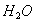is equal to 2 grams, and gram-atom of oxygen atom is equal to 16 grams. Gram-molecule of water is equal to 18 grams. Thus, one litre of water contains 1000/18=55.56 gram molecules of water.

As the mass of hydrogen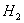in water molecule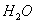is 2×100/18=11.1% and the mass of oxygen atom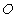is 16×100/18=88.9%, the ratio between quantity of hydrogen and oxygen is preserved in one litre of water as well. It means that 1000 grams of one litre of water contain 111 grams of hydrogen molecules  and 889 grams of oxygen atoms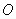.

One litre of molecular hydrogen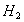has mass of  0.09 grams, one litre of molecular oxygen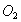has mass of 1.43 grams. It means that from one litre of water it possible to produce 111/0.09=1222.2 litres or 1222.2/22.4=54.56 moles of molecular hydrogenand 889/1.43=621.67 litres or 621.67/22.4=27.75 moles of molecular oxygen.

Let us pay attention to the fact that mass of hydrogen atom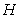is half mass of hydrogen molecule. As molecular volume of  all gases is similar and is equal to 22.4 litres, it means that one litre of water contains 111/0.045=2444.4 l or 2444.4/22.4=109.12 moles of atomic hydrogen .

Hydrogen atom fusion is  the process of junction of free proton with free electron. Energy of the photons emitted by the electron depends on energy level, on which the electron is held. If it is held on the first, non-exited energy level, prior to it the electron emits a set of the photons with energy of 13.6 eV, which is equal to the energy of hydrogen atom ionization. If the electron is held on the second level, energy of a set of the emitted photons is 3.4 eV; if it is held on the third level, energy is equal to 1.5 1itres eV; if it is held on the fourth level , energy is equal to 0.85 eV, etc. (s. Table 29).

The analysis shows that the electron of hydrogen atom can be on the first non-exited energy level only in the case if there are no external existing factors in the form of variables of the electric fields. If external disturbance is constantly present, the electron in the atom begins  to move from one energy level  to another energy level. In this case energy of the emitted photons and the absorbed ones will correspond to interlevel transitions of the electron .

Now let us consider the fusion process of water molecule fusion. It begins from the formation of hydrogen atom. When the electron is bound with the proton, it will try to occupy non-exited energy level and will emit the photons with total energy of 13.6 eV, equal to ionization energy of hydrogen atom. If we convert this energy into kilojoules (kJ), we shall have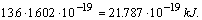(281)

When one mole of atomic hydrogen is formed, energy is released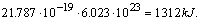(282)

At the temperature below 4500…5000°C hydrogen atoms  unite in molecules. As chemists think, energy released during this process is equal to 436 kJ per mole. When hydrogen molecule unites with oxygen atom, water molecule is formed with the release of energy of 285.8 kJ per mole. If one treats the above-mentioned values of energy being released consequently during the fusion of hydrogen atom, hydrogen molecules and water molecules, the following quantity of energy will be released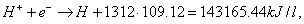(283)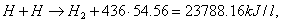(284)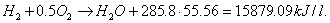(285)

If we sum up the results being obtained, we’ll have 182832.69 kJ per litre. It is potential energy, which can be released during the above-mentioned consequent fusion of one litre of water. If we take into account the fact that energy content of one litre of gasoline is 30000 kJ, it is 6 times less than the energy released during the formation of chemical bonds of one litre of water molecules beginning from the formation of atomic hydrogen .

Hydrogen mass obtained from one litre of water is equal to 1222.2×0.09=109.998 g. Energy content of one gram of molecular hydrogen is equal to 142 kJ, and energy content of hydrogen produced from one litre of water is 142×109.998=15619.72 kJ. It is half of  energy content of one litre of gasoline.

If hydrogen atoms were united in molecules being on the first energy levels, 182832.69 kJ per l of energy would be released during the fusion of one litre of water. But everything points out to the fact that hydrogen atoms unite in molecules only in exited state when their electrons occupy higher energy levels. A light zone to the right of a bright band on the spectrogram proves it (Fig. 82) .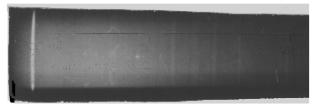Fig. 82. Spectral line (light line to the left) of the second energy level of hydrogen level with the light continuos zone (to the right from the line) being photographed by E.D. Zykov from plasma of the plasma – electrolytic reactor.

The bright band itself corresponds to energy of the photons emitted by the electrons during the transit from the third energy levels to the second energy levels, and the light zone to the right of this band corresponds to the spectrum of molecular hydrogen and demonstrates that the electrons of hydrogen atoms occupy higher energy levels before the formation of its molecule. As prior to the unification in molecules hydrogen atoms are in exited state, the energy of this state should be taken into account during the fusion of the atoms and later on of hydrogen molecules and water molecules. But we do not know a number of energy level of the electron in hydrogen atom yet, at which it unites with the neighbouring atom and forms hydrogen molecule.

One spectral line of hydrogen atom, which corresponds to the transition of the electron from the third energy level to the second one, is shown in the spectrogram (Fig. 82, to the left). Energy of the photon emitted by the electron during this transition is equal to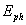=(12.0.87-10.198)=1.89 eV. The bright zone to the right from the band is the molecular spectrum of hydrogen .

We should note that the electrons of the atoms and the ions form on the spectrogram the distinctive bands, which correspond to the fixed energy levels. The molecules form the so called striped spectra, which are often merged into continuous light zones , . These are unfixed energy levels. Energies corresponding to fixed energy levels of the atoms and the ions are determined with great accuracy. Energy of unfixed  energy levels formed by the electrons of the atoms connected in molecules is changed in definite ranges. In some cases it is possible to determine an average value of energy corresponding to some ranges. For example, chemists have determined that 436 kJ are released during the fusion of one mole of hydrogen molecules. Let us try to determine, at which energy levels the electrons in hydrogen atoms are arranged before they are united in a molecule. Let us calculate the energy corresponding to  chemical bonds in hydrogen molecule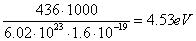As calculated per atom, it is equal to 4.53/2=2.26 eV. It is binding energy between the atoms in hydrogen molecule. It is not difficult to calculate  that 2.55 eV of energy are released during the transit  of the electron of hydrogen atom from the fourth energy level to the second one. If  one takes into account that the spectrum of hydrogen molecule (the light zone to the right from the bright band in Fig. 82) is formed in the zone before the second energy level, i.e. in the zone with energy, which is a bit less than energy of 2.55 eV, there is every reason to consider that prior hydrogen molecule formation the electrons of its atoms are on the fourth fixed energy levels. Binding energy of the electron with the protons is equal to 0.85 eV (Table 29).

Now let us give a variant of the calculation of energy, which is released during the fusion of one litre of water being the nearest one to reality. It corresponds to the case when the electrons of hydrogen atoms being born are kept at the fourth energy levels and then they are united into molecules. In this case during the formation of one hydrogen atom  energy of (13.598-12.748)=0.85 eV is released, or in the calculation per mole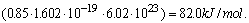(286)

Then the equation (286) gives this quantity of energy (82.0 x 109.12)=8947.84 kJ per litre, and the total quantity of energy is (8947.84+23788.16+15879.09)=48615.1 kJ per litre during the fusion of one litre of water. It is more than burning of one litre of gasoline (30000 kJ) or hydrogen (15879.09 kJ) obtained from one litre of water.

Thus, the variant of the fusion of hydrogen molecules is the most probable at the moment when the electrons of hydrogen atoms are on the fourth energy levels. In this case during the fusion of 1 litre of water energy is released, which is (48615.1/30000=1.62) 1.62 times greater than energy produced during burning of one litre of gasoline and is (48615.1/15895.15=3.0) 3.0 times greater than energy produced during burning of hydrogen produced from one litre of water.

Thus, in order to produce additional energy it is necessary to synthesize at first hydrogen atoms, then the molecules. The processes of their fusion  are the main sources of additional energy.

In an ideal case in order to check these calculations it is necessary to take free protons, to connect them with free electrons and to obtain atomic hydrogen and then molecular hydrogen. Then it is necessary to unite molecular hydrogen with atomic oxygen and to produce water. When energy released during the fusion process of hydrogen atoms, its molecules and water molecules is measures, it is possible to determine, which calculation method reflects reality more exactly. But it is difficult to carry out such ideal process. It is easier to find an economical way of water molecule distraction and to obtain additional energy with the help of its fusion in the above-mentioned sequence.

Now we see that additional energy is generated by the electrons. Where do they take it from? When we have considered the model of the electron, we have found out that it can exist in free state only in case when its electromagnetic mass is strictly determined. When it is combined with the atomic nucleus, it emits a part of energy in the form of the photons, and its electromagnetic mass is reduced. But stability of its state does not become worse, because energy taken by the photon is compensated by binding energy of the electron with the atomic nucleus. When it is separated from the atom and becomes free, it should restore its mass, which corresponds to its free state, in order to maintain its stability. Where does the electron take it from? The source is the only one - the physical environment in the form of ether. From this environment, it restores the energy (mass) being lost in the form of emitted photon. When the electron restores the constants (mass, energy, charge), it acquires stable free state.

When the conditions for the electron’s entry into bond are formed, it begins to emit energy in the form of the photons at once, which corresponds to binding energy. In a new stage of free state, it restores its constants (mass, charge, energy) again absorbing ether from the environment. Thus, the electron transforms energy of the environment into energy of the photons .

We have already published this hypothesis in one of our articles printed in USA . It is published in Russian for the first time.  A question arises: is free space available in the atom, which can serve as a source of ether absorbed by the electron in the process of restoration of its constants? The answer can be got from the geometrical parameters of the atom, and they are as follows: if the size of the atomic nucleus were equal to one mm, the size of one electron in the atom would be nearly one metre, and the size of the atoms itself would be nearly 100 metres. It means that the atoms has enough space filled with ether, which is necessary for the electron for the restoration of its constants after the bond with  an atomic nucleus or with the electron of the neighbouring atom is lost.

It means that physical vacuum is a source of additional energy and the electron is a converter of energy of vacuum into energy of the photon .

The given results of calculations and experiments demonstrate the possibility of  production of additional energy during water electrolysis, but for this purpose it is necessary to create the conditions for the implementation of this possibility.

The preliminary analysis of manifestation of additional energy in the phenomena of water cavitations shows that the source here is the same as during water electrolysis. Mechanical destruction of water molecules leads to the further fusion of the atoms and the molecules of hydrogen and water. The electrons play here the same role as during water electrolysis. They transform energy of vacuum into energy of the photons.

11.5. Clusters and their Binding Energies

It is known that water molecules can be units with each other forming the associations called clusters. The clusters are a set of the molecules of the same name, which are  connected with each other by hydrogen bonds, as it has been considered earlier. And it is so indeed. Water molecules connect the protons of the hydrogen atom into clusters (Fig. 83).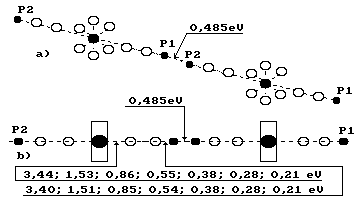Fig. 83. Diagram of a cluster made of two water molecules

Now we can call  them electron bonds, proton bonds or electron - proton bonds. The chemical formula of the cluster consisting  from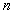of  ions of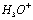and   water molecules is put down in the following way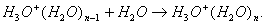(287)

If ion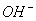participates, the reaction takes place in such a way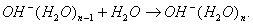(288)

There are experimental data of binding energies between water molecules and ions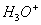andwhen their different quantity is present in a cluster (Table 37) .

Table 37. Values of binding energy in clusters, eV

 Value n 0-1 1-2 2-3 3-4 4-5 5-6 6-7 H3O+ (H2O)n 1.56 0.97 0.74 0.67 0.57 0.51 0.45 OH-(H2O)n 1.1 0.71 0.66 0.62 0.61 - -

For example, 5.47 eV  are consumed  for the formation of cluster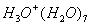when n=7.

The process of the formation of clusters is an endothermic one, i.e. during the formation of the clusters the electrons, which unite the molecules with each other, move off from the atomic nuclei in their cells.

Having the structure of water molecule (Fig. 72-74) we see other possibilities of cluster formation. There are no restrictions for the formation of proton-to-proton bonds between water molecules. The protons of the first hydrogen atoms in two water molecules uniting with each other form the association of two molecules (Fig. 83). The protons of the second hydrogen atoms in water molecules as well as the first and, probably, the second electrons of oxygen atoms can be involved in this process. As a result, the number of molecules in the cluster will be increased.

Thus the presence of the ions of hydroxyl and hydroxonium is unnecessary for the formation of the clusters of water. Let us consider the structure of parahydrogen molecule in Figs 53, b and 80, c. It can be a connecting link in the cluster, and after its destruction hydrogen can be born at once not in the atomic state, but in the molecular one.

If under usual conditions water molecules are united in the associations known as clusters, binding energy between clusters moves to zero during the conversion into vaporous state, and we have the possibility to calculate binding energy between the molecules in the cluster at the temperature of 20°C. We’ll use vapour formation energy of 2595.2 kJ per kg for this purpose. Let us convert this energy into electron – volts in the calculation per molecule (Fig. 83).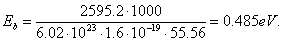(289)

This result  is close to binding energy (0.54 eV) of the electron of the first hydrogen atom in water molecule when it is on the fifth energy level (Table 35) and proves that the proton of this atom spends the majority of magnetic line of force for the bond with the electron, and the minority is free and can be used for the bond with the proton of hydrogen atom of the neighbouring water molecule (Fig. 83). One more problem takes place here, about which we’d like to say some words.

What law controls the alternation of the  magnetic poles of the protons in atomic nuclei? The reader understands that the answer to this question can be given by a new book, and not only by one book, that’s why we give a short answer, which seems to be obvious. It is impossible that the south or north magnetic poles of all protons of the nucleus are directed to its surface or to the centre. They alternate in such a way that strength of the nucleus is increased.  They are in sequence, and strength of the nucleus is increased. It can be easily seen that the axial neutrons and protons in the nucleus of the oxygen atom (Fig. 28) are connected by unlike magnetic poles at different ends of the nuclei axis and leave different magnetic poles.

It leads automatically to various magnetic polarity of the protons of hydrogen atoms in water molecules, and in this case the conditions are formed for proton-to-proton bond between water molecules and for the formation of clusters in such a way.

There is every reason to believe that one and the same proton in various nuclei of atoms can be arranged in such a way that the north magnetic pole in one nucleus will be directed to the centre of the nucleus and in other nucleus to its surface. Such arrangement of the magnetic field of the proton provides the formation of proton-to-proton bond between water molecules during the formation of clusters.

The atomic nuclei are the beginning of the formation of various magnetic poles of valence electrons on the surface of the atoms, that’s why there is every reason to believe that all atoms are divided into two classes according to this feature, these classes can be conditionally called «masculine» and «feminine».

If we imagine a cluster consisting of two water molecules, which have the forms of the balls with the diameters of 100 metres, the protons arranged on the surface of these balls and uniting them in a cluster have millimetre dimensions. The smallest, even mechanical influence will destroy this system creating the conditions for fluidity of water molecules.

If the clusters had been formed by electron - to - electron bonds, they would have had metre dimensions of the surface of one hundred metre molecules.

Now it is possible to calculate energy spent for heating of one water molecule by one degrees. It is known that 335.2 kJ of energy  is spent for heating of one litre of water from 20°C to 100°C. Calculating per molecule it will be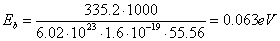(290)

It is energy value, by which binding energy of valence electrons is changed  in water molecule if it is heated from 20°C to 100°C. If we divide 0.063 eV by 80, we’ll get a value, by which binding energy of valence electrons is changed during water heating by one degree. It is equal   0.00078 eV. This energy corresponds to the photons of a  relic range, which covers a part of the infrared range and the microwave one (Table 34).

Thus, minimal energy of the photons absorbed by the electrons of water molecules during heating corresponds to the energies of the photons of the relic range; it serves as an indirect proof that this range a boundary of existence of  single photons .

Now it is possible to specify energy level number, at which the electrons of hydrogen atoms are in water molecules. For this purpose let us convert energy (286 kJ) of fusion of one mole of water in electron - volts.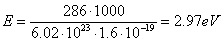.                                               (291)

It will be 2.97/2=1.485 eV in calculation to one bond. It is near to energy of 1.51 eV of the third atomic energy level. Later using energy consumption (4 kWh) for the production of one cubic metre of hydrogen we have found out that binding energy of hydrogen atom with oxygen atom in water molecule is equal to 1.59 eV. Energy consumption for heating of water during its electrolysis is included into this value. If water is heated up to 80°C, binding energy is equal to (1.59-0.00078x60)=1.543 eV at 20°C. Good approximation of two results to energy (1.51 eV) of the third atomic energy level of hydrogen atom points out to the fact that we can trust the fusion energy values of one mole of molecular hydrogen of 436 kJ per mole and fusion energy of one mole of water molecule of 286 kJ per mole. The results being obtained show that the electron of hydrogen atom in water molecule is not on the third atomic energy level exactly (1.51 eV), but near it.

One cubic metre contains 1000 x 0.09=90 g of hydrogen. Energy content of one cubic metre of hydrogen is 142 x 90=12780 kJ. Produced energy of 12780 kJ is equal to (12780/3600)=3.55 kWh. If one can manage to spend less energy consumption for the production of one cubic metre of hydrogen than 3.55 kJ, it will be a competitive energy carrier .

The analysis of the model of the electron (Fig. 18) shows the possibility of the formation of the clusters of the electrons. The unlike magnetic fields of the electrons bring them together, and the like electric fields restrict this approach. Accuracy of this consequence is confirmed by the experiments , , .

The Foundations of Physchemistry of Microworld

Ó2003 Kanarev Ph. M.

Internet Version - http://book.physchemistry.innoplaza.net

<< Back to Physchemistry Book Index